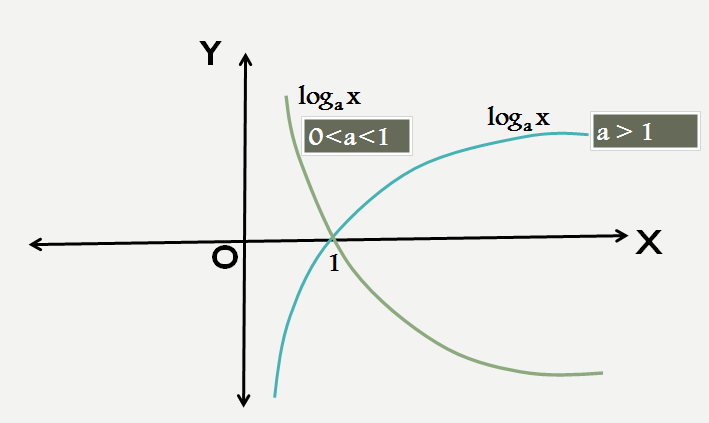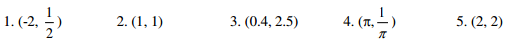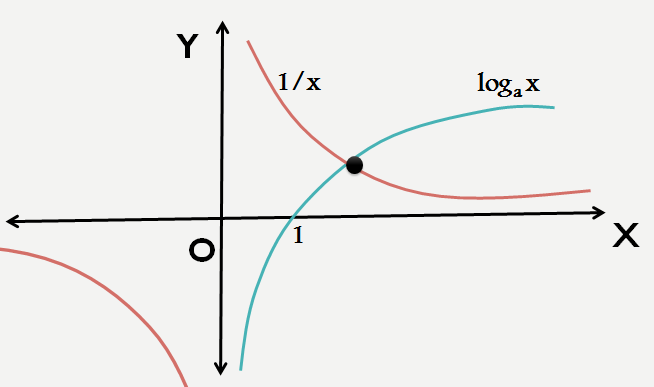# Algebra

#### FUNCTIONS-1

Logarithm: 1

Logarithm

Logarithm is an important chapter in Algebra and is the reverse of the process of exponentiation which raises an exponent of a number. Here logarithm of a number is defined to be the exponent to which a fixed number called as the base must be raised to get that number.

For example, we know that 23 = 8, so the logarithm of 8 with base 2 is 3, i.e. the exponent to which 2 (base) must be raised to generate 8 is 3, written as log28 = 3.

Similarly log4 8 would mean the exponent to which 4(base) must be raised to generate 8 i.e 3/2 or 43/2 = 8

So, in general if ay = x   {Exponential Form}

Then y = logax  {Logarithmic Form}

Here note that x must always be positive while the base (a) must always be positive and its value cannot be equal to 1.

Graph of LogAs one can see that log is not defined for any negative value of x or for x =0. So, one cannot find its curve on the negative X axis. Also, log takes values from  -∞ to +∞. It cuts the X axis at the point (1,0), so log1 = 0 irrespective of the base we are working on.

Properties of Logarithms:

a. log(m*n) = logm + logn

b. log(m/n) = logm – logn

c. log(mp) = plogm

d. logab = 1/logba

e. logab = loga/logb

f. log(am)bn  = (n/m)logab

g. alogab = b

If base is 10, it is known as common logarithm while if the base is ‘e’, then the logarithm is known as natural where ‘e’ is an irrational number whose value is between 2 and 3.

Example 1: If the value of log102 = 0.3010 and the value of log103 = 0.4771, then find the value of log812.

Solution: log812 = log(23)(22*3) = 1/3 (log2(22*3) = 1/3 (log222 + log23)

= 1/3 (2log22 + log23) = 1/3 (2+ log23)

log23 = log103/log102 = (0.4771/0.3010) = 1.585

log812 = 1/3 (2+1.585) = 1.195

Example 2: If log y x = (a log z y) =

(b log x z) = ab, then which of the following pairs of values for (a, b) is not possible?   (CAT 2006)Solution: log y x = ab  or logx/logy = ab   (1)

(a log z y) = ab    alogy/logz = ab  (2)

(b log x z) = ab     blogz/logx = ab  (3)

Multiply (1),(2) and (3) we get

Ab = (ab)3     or ab(ab-1)(ab+1) = 0

So, either ab = 0  or ab = 1 or ab = -1

All the options except for (2,2) doesn’t satisfies the above equations.

Example 3: If log10x – log10= 2 log x10, then a possible value of x is given by:

1. 10               2. 1/100           3.1/1000      4. None of these  (CAT 2004)

Solution: log10x – log10x = 2 log x10

log10x -1/2 log10x = 2logx10

½ log10x  = 2logx10

log10x = 4logx10

log10x = 4/log10x  or (log10x)2 = 4

log10x = 2 or -2   thus x = 100 or 1/100

Example 4:  If 1/3 log3M + 3log3N = 1+ log0.0085 then:

A. M9 = 9/N   B. N9 = 9/M         C. M3 = 3/N           D. N9 = 3/M   (CAT 2003)

Solution: 1/3 log3M + 3log3N = 1+ log0.0085

log3M1/3 + log3N3 = 1 + log(1/125)5

log3(M1/3*N3) = 1+log(5-3)5

log3(M1/3*N3) =1 -1/3log55

log3(M1/3*N3) =1-1/3= 2/3

32/3 = M1/3N3

Cubing both sides

9 = MN9

N9 = 9/M

Example 5: Of log32, log3(2x -5), log3(2x – 7/2) are in arithmetic progression, then the value of x is equal to    (CAT 2003)

A. 5               B. 4 C. 2                  D. 3

Solution:

As the terms are in A.P. so,

2log3(2x -5) = log32 + log3(2x – 7/2 )

Log3(2x -5)2 = log3{2*(2x -7/2)}

Comparing both sides we get

(2x -5)2 = 2(2x – 7/2)

Ony x = 3 satisfies the above equation

So, option (D) is correct.

Number of digits in a number

Logarithms are a useful tool to calculate the number of digits in a number. Let’s understand how.

Log101 = 0   log1010 = 1    log10100 = 2 , log101000 = 3 and so on

Now, if we are to find log107, then 7 lies between 1 and 10 , so value of log7 would something be between 0 and 1.

Similarly log 23 would be between 1 and 2 as  10 <23 <100

2< log297 < 3   as 100 < 297 < 1000

So, from above we can say that

log(N digit) would be N-1 point something.

Example 6: Find the number of digits in 297

Solution: Let y = 297

Taking log on both sides, we get

log10y = log10297 = 97*log102 = 97*(0.3010) = 29.197

As log(N digit number) = N-1 point something

So, y has 30 digits.

Example 7 When the curves y = log10 x and y = x -1 are drawn in the x-y plane, how many times do they intersect for values x 1?  (CAT 2003 Leaked)

1. Never         2. Once     3.Twice         4.More than twice.

Solutions: Drawing the graphs of logx and y = 1/x we getSo, there is only one point of intersection at the point will be 2 < x <3.

Example 8 : If log2[log7(x2 –x +37)] =1 , then what could be the value of x?

1. 3                  2. 5         3. 4 4. None of these

Solution:  Given : log2[log7(x2 –x +37)] =1

Log7(x2 –x +37) = 2

72 = x2 –x +37   or x2 – x -12 =0

x2 – 4x + 3x -12  = 0

x = 4  or x = -3

Thus, one of the value of x is 4, so option (3) is correct.

Example 9 : Find the value of x if

log(1/√2)(1/√8)= log2(4x +1)* log2(4x+1 +4)

Solution: L.H.S. = log(1/√2)(1/√8) = log(1/√2)(1/√2)3 = 3

So, 3 = log2(4x +1)* log2(4x+1 +4)

3 =  log2(4x +1)* log2{4(4x+1)}

3 =  log2(4x +1)*{ log2(4) + log2(4x +1)}

Let log2(4x +1) = t

3 = t(2 +t)

t2 +2t -3 = 0 or t = 1 or t = -3

log2(4x +1) = 1  or log2(4x +1) = -3

The second equation does not give a real value of x

So log2(4x +1) = 1   or 4x + 1 = 2   or x = 0